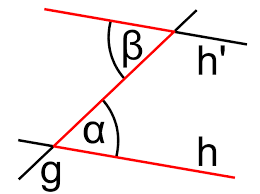# GCSE / National 5 Maths Glossary

## Acute angle

An angle less than a right angle (ie less than 90°). See also obtuse angle, reflex angle and right angle.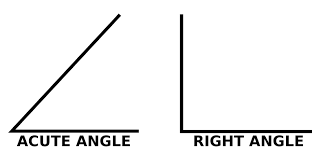Next to.

## Algebra

Where letters are used to represent an unknown number.

## Angle

An angle is the rotational difference between two lines, normally measured in degrees.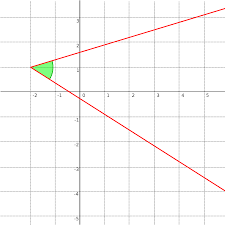## Approximate

A value close to the actual value of a number

## Arc

Part of a circle's circumference.## Area

The amount of skin a shape has.

Not symmetrical

## Average

One number that represents all the numbers in a set. Average is often used to mean the mean but median and mode are also averages.

## Axis

One of the scales used to represent a point in a coordinate system. For a 2D graph, there's an x-axis and a y-axis, for a 3D graph there's also a z-axis.

## BIDMAS

A mnemonic to help you remember which order to carry out operations: Brackets, Indices, Division, Multiplication, Addition and Subtraction.

Doing operations in a different order makes the answer wrong. eg 2+1x3=5 but (2+1)x3=9

## BODMAS

A mnemonic to help you remember which order to carry out operations: Brackets, Order, Division, Multiplication, Addition and Subtraction

Doing operations in a different order makes the answer wrong. eg 2+1x3=5 but (2+1)x3=9

## Base

The bottom side of a triangle.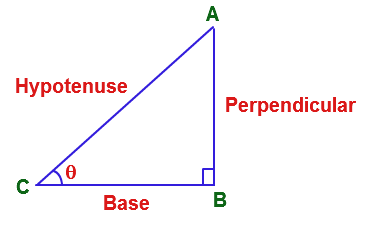## Bearing

A three digit angle measured clockwise from north, eg 030°

## Bisect

To cut exactly in half

## Brackets

The part of an expression that must be carried out first. eg 2+1x3=5 but (2+1)x3=9

If there is a bracket inside another bracket, start with the innermost bracket.

## Calculate

Work out a value. Calculate does not mean you need to use a calculator

## Centilitre cl

A measure of volume. Centi- means a hundredth, so there are a hundred centilitres in a litre. One litre is 1,000 cm3. You can think of this as a shape measuring 10cm x 10cm x 10cm.

## Centimetre cm

A measure of distance. Centi means a hundredth, so there are 100cm in one meter. Things that are 1cm include: the width of a staple, the thickness of a note pad and the diameter of a belly button.

## Centre of enlargement

The point around which scaling is defined. If your pencil was scaled by a factor of 3, it would be 3 times as long. If the centre of scaling was 1m to the left, then the scaled pencil would move 2 metres to the right because it is now 3m from the centre of enlargement.

## Centre of rotation

The point that did not move when a shape is rotated. For a wheel the centre of rotation would, of course, be the centre of the wheel.

## Chord

A straight line between two points on the circumference of a circle.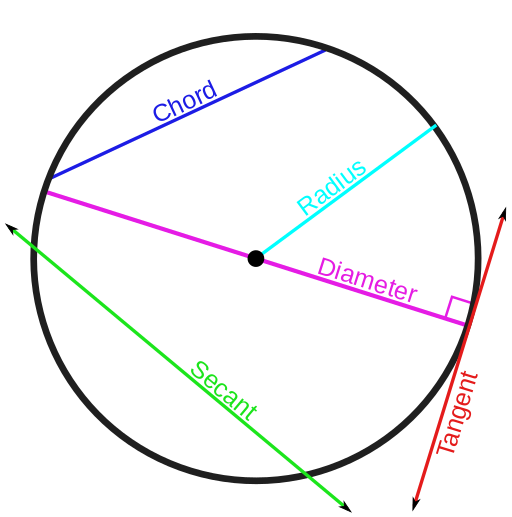## Circumference

The perimeter of a circle.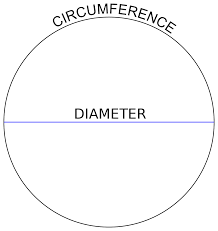## Class width

The range of values (ie upper boundary minus lower boundary) represented by a group.
 Age range Class width Frequency 4-7 3 4 8-11 3 6 12-15 3 7 15-18 3 5

## Coefficient

A number that a variable is multiplied by. eg the coefficient of 3x is 3.

## Congruent

You can place one shape exactly on top of a shape that's congruent with it. You may need to rotate it, translate it and/or reflect it.

## Constant

A number that is always the same, represented by a symbol or a letter. One example is π (the ratio of a circle's diameter to its perimeter). Another example is c, the speed of light, used in E=mc2.

## Convention

The normal way of doing things

## Credit

Add money to a bank account.

## Cross section

The shape when you slice a 3d shape along its length

## Cube

A 3D shape where every surface is a square.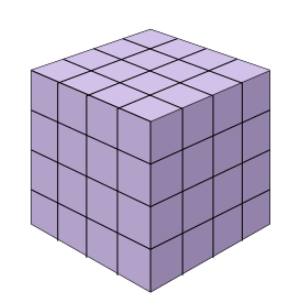## Cube number 3

A number which is the result of multiplying a whole number by itself, twice

eg4 cubed = 43 = 4 x 4 x 4 = 16 x 4 = 64

## Cubed 3

A number which is the result of multiplying a whole number by itself, twice

eg4 cubed = 43 = 4 x 4 x 4 = 16 x 4 = 64

## Cuboid

Like a cube but some sides are rectangles rather than squares.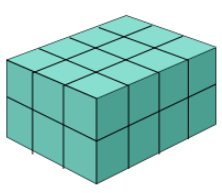## Cumulative frequency

A running total of frequencies. eg
 Age range Frequency Cumulative Frequency 4-7 4 4 8-11 6 10 12-15 7 17 15-18 5 22

## Day

A time period of 24 hours, normally measured from midnight to midnight. There are 7 days in a week and 365 ¼ in a year (365 years in most years, but 366 in a leap year)

## Debit

Take money out of a bank account

10 sided polygon

## Decimal

A number that is not a whole number, expressed with a '.' in it, rather than as a fraction. eg 3.14 , 2.7

## Decimal places

The number of digits after the decimal point.

## Decrease

To make a quantity smaller

## Degrees

Literally a measure of something. It is commonly used to mean either:
1. A measure of temperature, either as degrees centigrade or degrees farenheit
2. A measure of an angle. A circle is divided into 360 degrees. Why 360 and not 100? Because 360 has a lot of factors.

## Denominator

The bottom part of a fraction. numeratordenominator or dividenddivisor

## Describe

To use a mathematical function to convey the properties of something.

For example: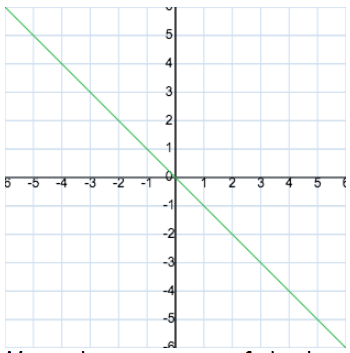The green line above is decribed by the function y = - x

It is also described by x = - y but by convention, we write the y to the left of the equals sign.

## Diameter

The straight line length passing through the centre of a circle from one point on a circumference to an opposite point on a circumference.## Difference

Subtract the smaller value from the larger value. ie the difference between 5 and 2 is 3.

## Distance

How far away something is. eg at its closest, the distance to the moon is 360,000km .

You also see distances (in miles) on road signs.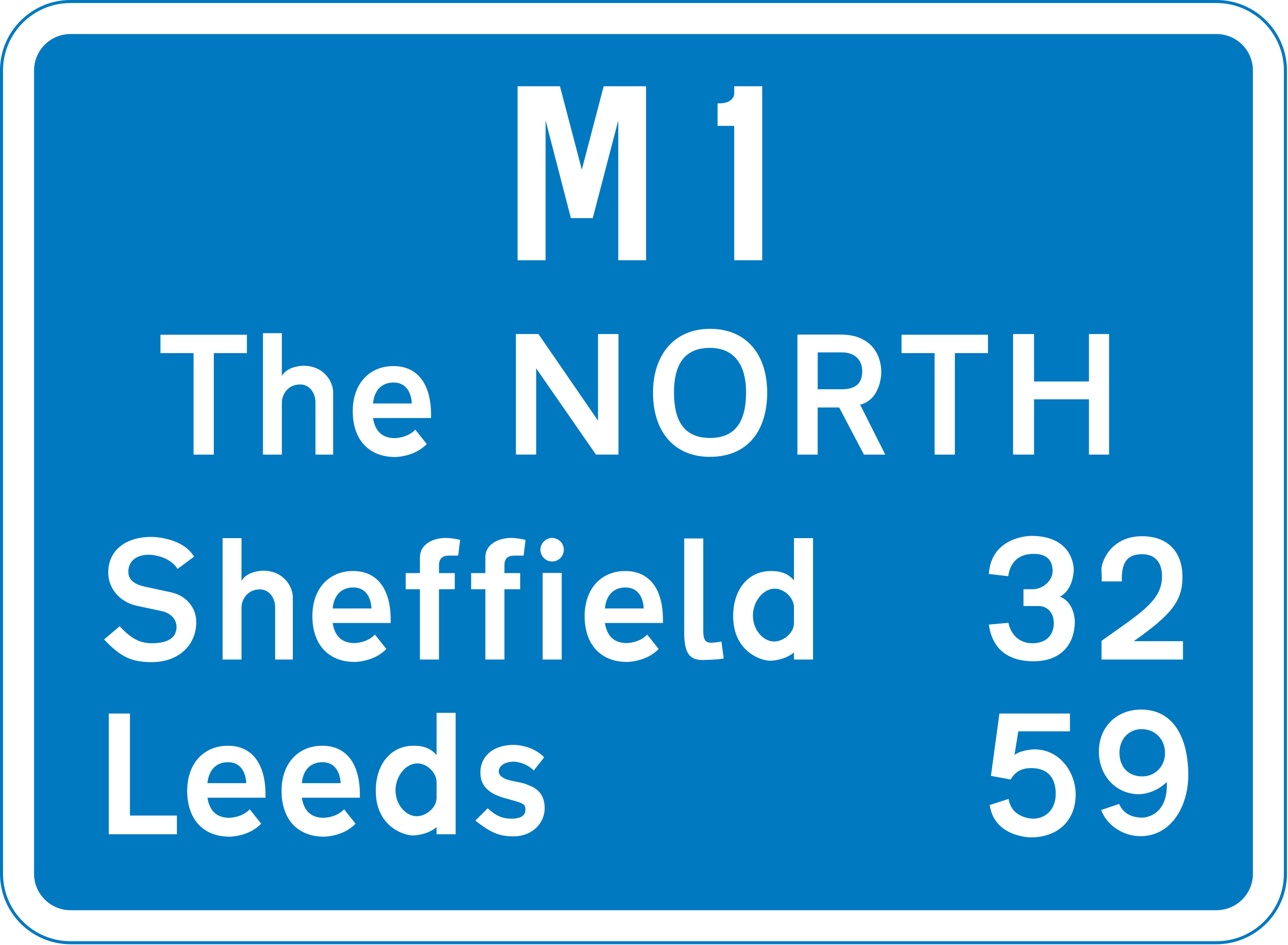## Distribution

How data is scattered. This can be represented by numbers (ie standard deviation) or as a plot.

## Divisor

The bottom part of a fraction. numeratordenominator or dividenddivisor

## Dvidend

The top part of a fraction. numeratordenominator or dividenddivisor

## Equal

Used to show that one quantity has the same value as another

## Equation

A statement showing one expression has the same value as another. eg 2+3=7-2

## Equilateral

A shape where all sides have the same length.

This could be an equilateral triangle, which is also a regular triangle...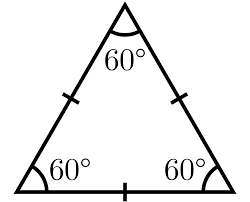...or an irregular equilateral octagon, for example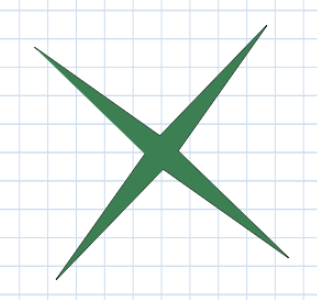## Equilateral triangle

A regular triangle. Equilateral or equi(equal) lateral(side) means all sides have the same length as each other. In the case of a triangle, if the side lengths are the same, the angles will also be the same as one another.

Mathematicians don't like to 'overspecify' things. They (ok, we) like to give a tiny bit of information from which you can imply the rest of what you might want to know.## Estimate

To find an approximate answer to a problem. This often involves solving the problem with easier numbers. ie 10.2 x 4.8 ≈ 50

## Even

A number which is a multiple of 2. All even numbers end in 0, 2 , 4 , 6 or 8. Whole numbers that aren't even are odd. They're not strange; that's just what they're called.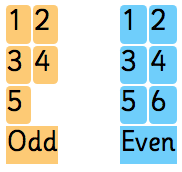## Even number

A number which is a multiple of 2. All even numbers end in 0, 2 , 4 , 6 or 8. Whole numbers that aren't even are odd. They're not strange; that's just what they're called.## Expand

To multiply out brackets. eg 2(x+3) expanded becomes 2x+6.
Or (x+1)(2x+3) = 2x2+5x+3

## Expression

A collection of terms which can contain variables (letters) and numbers, eg 2x+y=8

## Factor

A number that fits exactly in another number. eg 6 is a factor of 12

## Factorise

The opposte of expand. Take a common factor out of an expression to put it into brackets. eg 2x+6 factorised is s(x+3)
Or 2x2+5x+3 = (x+1)(2x+3)

## Figures

Numbers. Fifty two in figures (or numerals) is 52.

## Formula

An equation that describes the relationship between two or more variables.

## Frequency

The number of times something occurs. It's a tool to make data more helpful.

For example a dodgeball club may have the following members:
 Age range Frequency Cumulative Frequency 4-7 4 4 8-11 6 10 12-15 7 4 17 15-18 5 22

## Frequency density

A mesure of how many values a class represents. It is calculated as frequency divided by class width.

## Function

One or more mathematical operations carried out on the input. For example, if the function is 2x +1, and the input (x) is 2, the output would be 5 (2 × 2 +1 )

A measure of how steep a line is found by dividing the distance up by the distance across.. You see this expressed as a percentage (ie multiplied by 100) on road signs: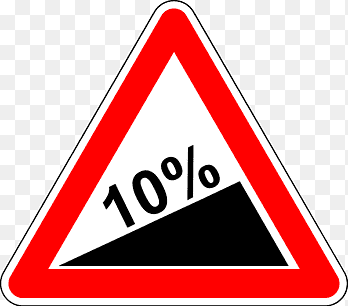## Gram g

Gram is a measure of mass. 1g is about the weight of a small paperclip or a plastic pen cap. 1 litre of water weighs 1,000g (= 1kg)

## Gramme

Gram is a measure of mass. 1g is about the weight of a small paperclip or a plastic pen cap. 1 litre of water weighs 1,000g (= 1kg)

## HCF

The Highest Common Factor (HCF) is the largest factor common to a set of numbers. eg the HCF of 12, 24 and 32 is 4

## Heptagon

A heptagon (also known as a septagon) is a 7 sided polygon (shape).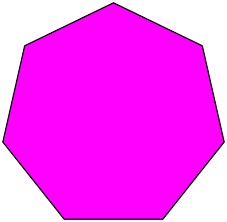Is a 50p coin a septagon (or heptagon)?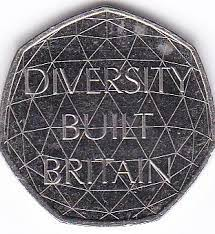## Hexagon

A six sided polygon (shape).

A hexagon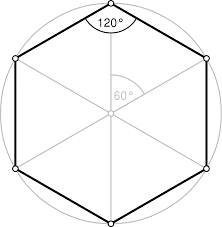Hexagons with friends: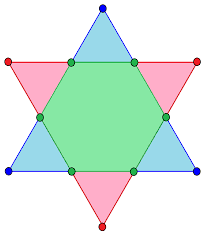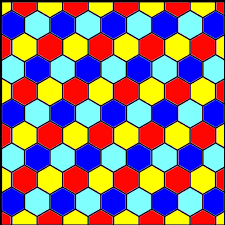## Highest Common Factor

The Highest Common Factor (HCF) is the largest factor common to a set of numbers. eg the HCF of 12, 24 and 32 is 4

## Histogram

Like a bar chart except each bar represents a range of data points. The width of each bar is proportional to the class interval and the area is proportional to the frequency.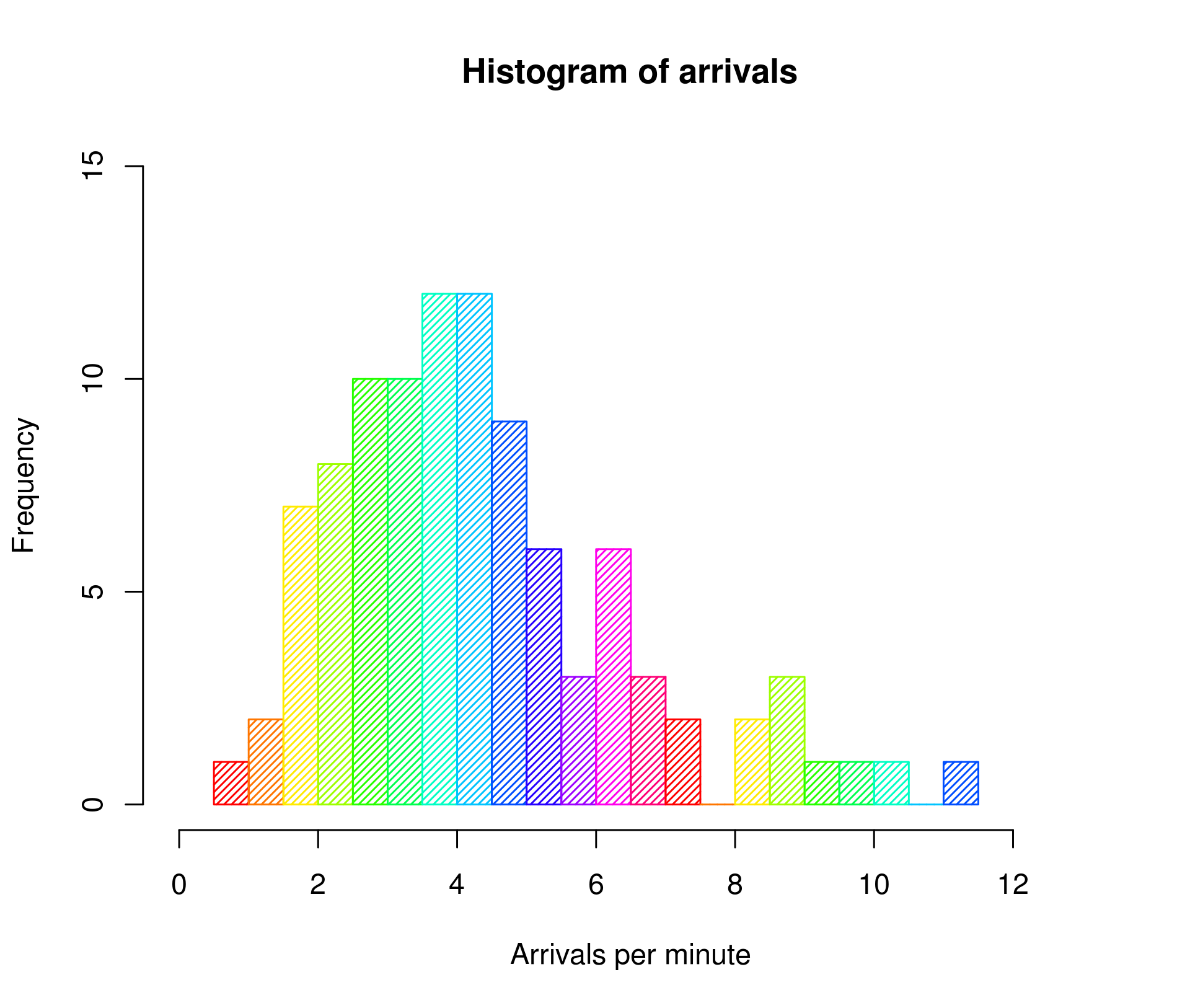## Horizontal

A 'flat' orientation. The surface of water is always horizontal.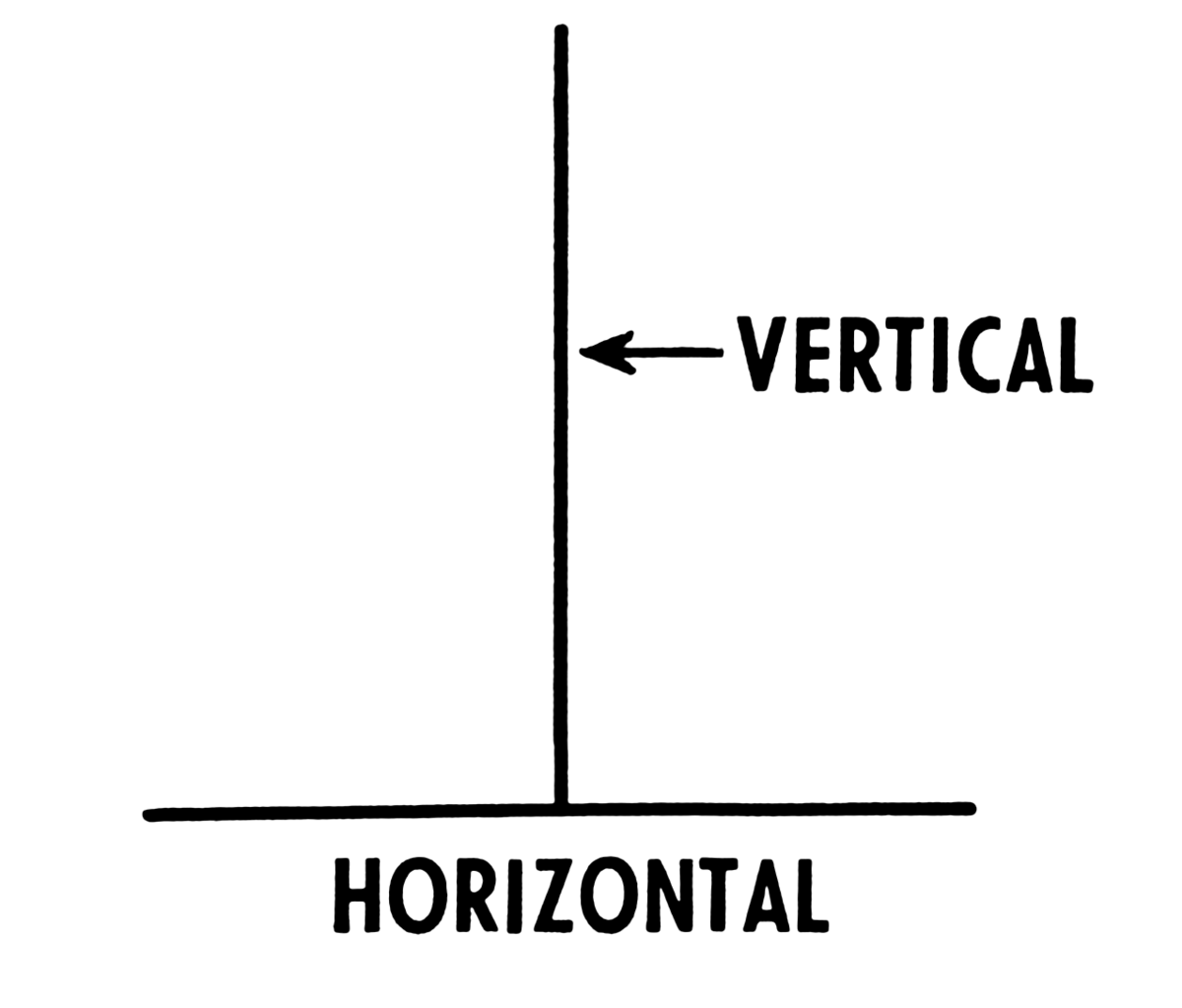## Hypotenuse

The longest side of a right angled triangle.This is essential to know for pythagoras.

## IQR

The Inter Quartile Range (IQR) is the difference between the upper and lower quartile.## Increase

To make a quantity larger.

## Indices 4

eg 24 - 4 is the indice or power

Whole number.

## Inter-quartile range

The Inter Quartile Range (IQR) is the difference between the upper and lower quartile.## Irrational

Irrational numbers are never ending and not recurring decimals that can't be written as a fraction of integers.

17 is never ending and not recurring but can be written as a fraction so is not irrational.

√2 is irrational.

## Irregular

A polygon that is not regular. ie its sides are not all the same length and/ or its angles are unequal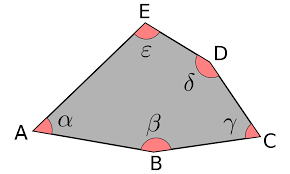## Iscosceles

An iscosceles has two sides the same shape. It is often said of triangles.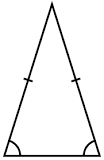But can also be said of other shapes: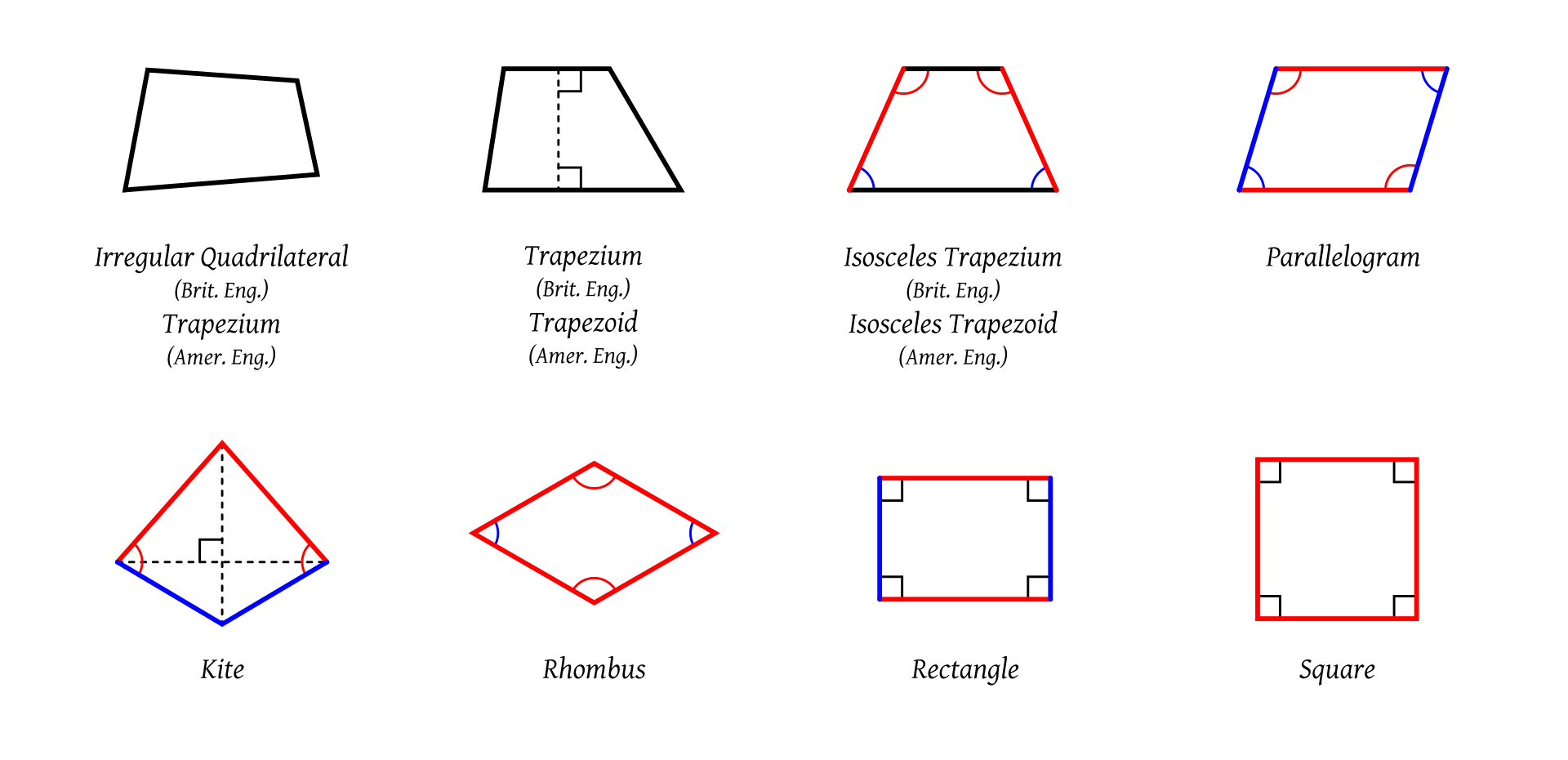## Kilogram kg

The SI unit of mass. About the same mass as a bag of sugar. 1kg is 1,000g

## Kilometre km

A measure of distance. 1Km=1,000m

## Kite

In maths, a kite is a quadrilateral with one line of symmetry stretching between two opposite corners. If there is another line of symmetry between the other two corners, it's a rhombus.## LCM

The LCM is the smallest multiple common to a set of numbers. The LCM of 6 and 4 is 12. For prime numbers, the LCM will be the product of the numbers.

## Leap year

In a leap year there's an extra day, so there are 366 days in a leap year, instead of 365. Every 4th year is a leap year.

The reason for it is to keep summer in the summer months and winter in the winter months.

## Length

The longest dimension of an object that is not its height..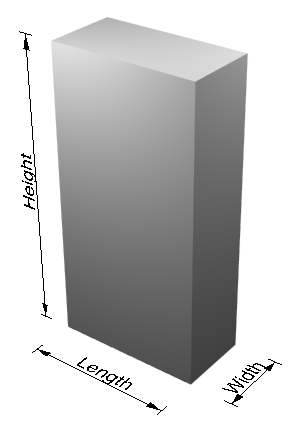## Line of symmetry

A line (or axis) of symmetry is a lline such that what's on one side of the line is mirrored by what's on the other side of the line

A square has 4 lines of symmetry, cutting it in half along the edges, or cutting it in half across its corners.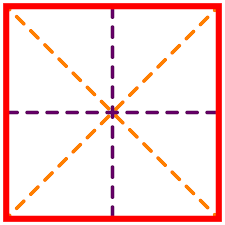## Litre l

A measure of volume. One litre is 1,000 cm3, so the volume of 10cm x 10cm x 10cm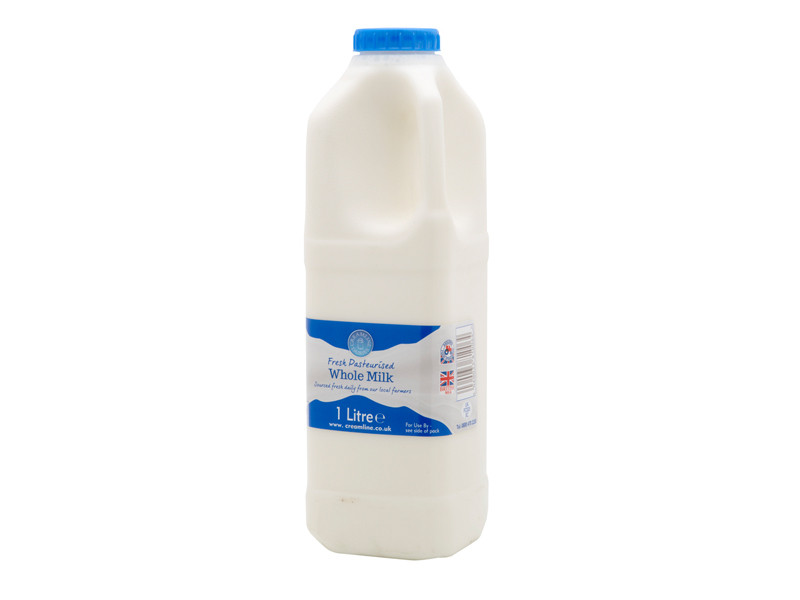## Loci

Loci is the plural of locus. Most formulas you encounter have only one locus. An example of a formula with many loci would be a tangent plot (ie y=tan(x)).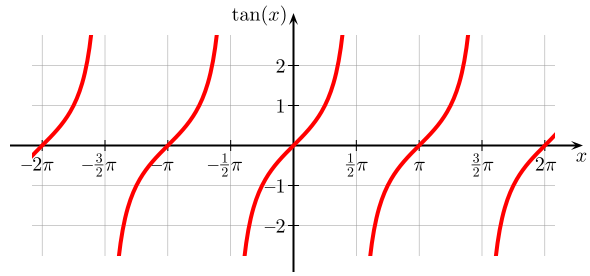## Locus

A locus is a set of points which satisfy a condition (such as a formula). Most commonly the points form a line.

eg, the green line represents the locus of y = - x.## Lower range

The smallest value in a data set.

## Lowest Common Multiple

The LCM is the smallest multiple common to a set of numbers. The LCM of 6 and 4 is 12. For prime numbers, the LCM will be the product of the numbers.

## Mean

The most common type of average, formed by adding up the data set and dividing by the number of items.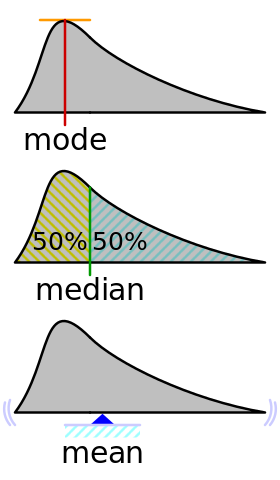## Median

A type of average: the middle value when the data set is sorted form smallest to largest.## Metre m

A measure of distance. 1km =1,000m, 1,000mm =1m. Some things that are about 1m: the length of a guitar, the width of a door frame, the height of a kitchen counter.

## Millilitre

A measure of volume 1cm cubed, or one thousandth of a litre. A teaspoon is about 5ml.

## Millimetre

A tiny measure of distance. There are 1,000mm in 1 metre. Things that are 1mm include: a pencil tip, a mustard seed, a sewing needle tip, a grain of sand.

## Modal

Relating to the mode (average). You could say the mode in a set of human height data is 178cm, or the modal height is 178cm.

## Mode

The most commonly occurring value in a data set. You can remember it as it's the same word used in fashion a la mode.

If there are two (or more) equally common values, there would be two (or more) modes.## Month

A time period dividing the year into 12. There are between 28 and 31 days in a month. You can remember them by the rhyme:

30 days hath September, April June and November
All the rest have 31
Except February all alone with 28 or 29 in a leap year.

## Multiple

A number that's been multiplied by another number. eg 6 is a multiple of 2 (and also a multiple of 3)

## Natural number

A positive integer.

If you were counting sheep and came up with half a sheep or a negative number of sheep, that would be unnatural. Zero is not a natural number either. A shepherd with zero sheep is not a shepherd.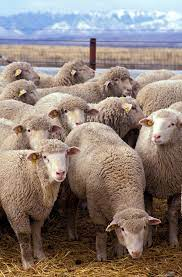## Negative

A number less than zero.

The temperature in a normal household freezer is about -20°c. Negative numbers are also seen around money.

Not 1.

## Non-unitary fraction

A non-unitary fraction is a fraction that has a numerator that is not 1.

14 is a unitary fraction.

34 is a non-unitary fraction.

24 is also a non-unitary fraction, even though it can be simplified to a unitary fraction.

## Nonagon

A 9 sided polygon.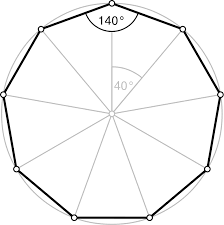## Numeral

Numbers. Fifty two in figures (or numerals) is 52.

## Numerator

The top part of a fraction. numeratordenominator or dividenddivisor

## Obtuse angle

An angle between 90° and 180 °.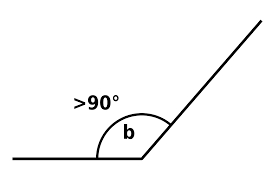Angle b is obtuse.

## Octagon

An 8 sided polygon.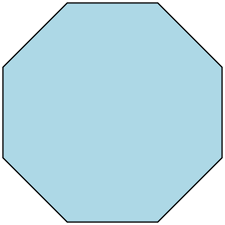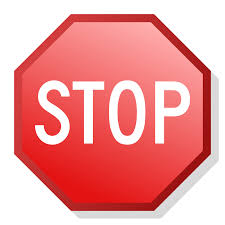## Odd number

A number that is not even. Odd numbers always end in 1, 3, 5, 7, or 9.## Operation

A simple function, most commonly addition, subtraction, multiplication or division.

## Orientation

The direction something is facing.

## Parallel

When two or more lines are parallel they are always the same distance apart. In other words the lines are aligned in exactly the same direction.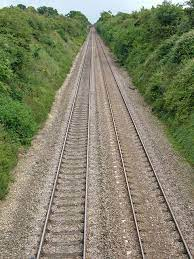What would happen if train tracks weren't parallel?

## Parallelogram

A quadrilateral where each side is parallel to its opposite side. A rectangle is a special parallelogram.## Pentagon

A 5 sided polygon.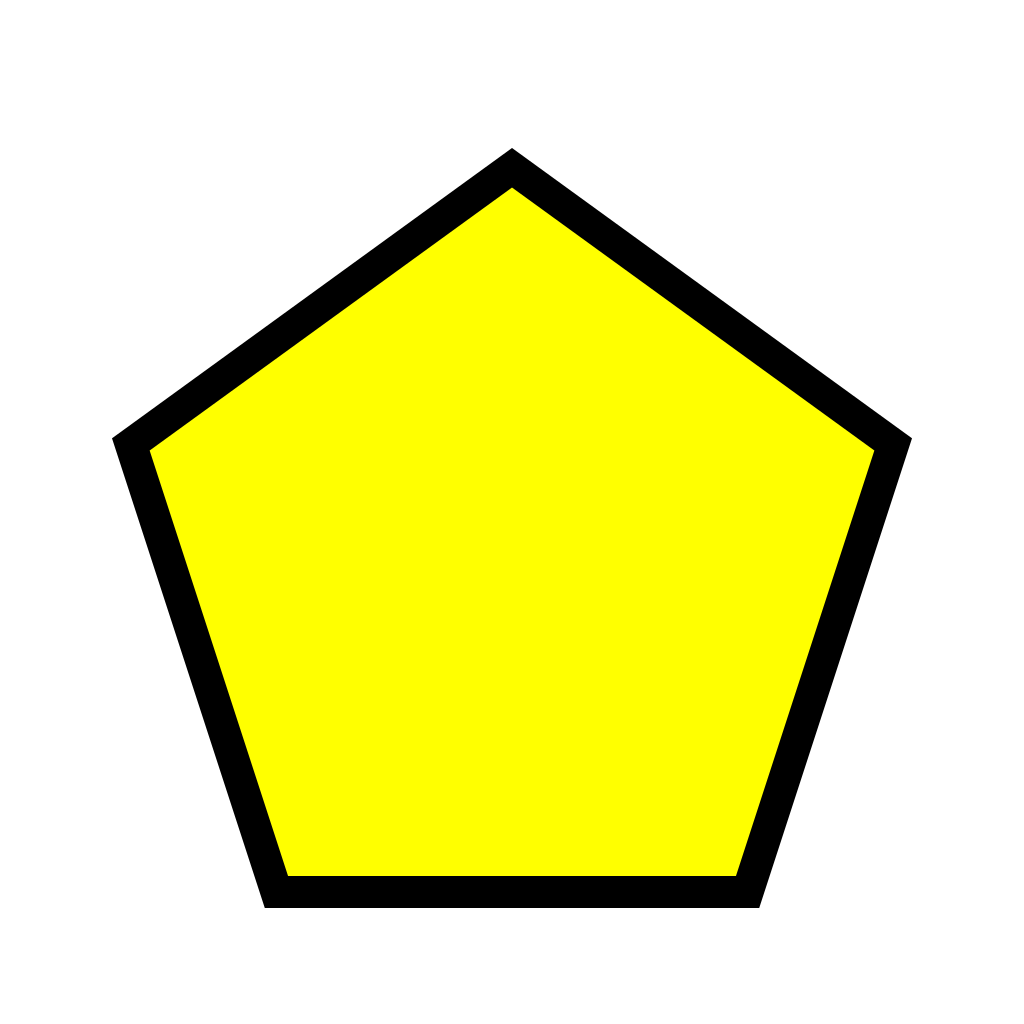## Perimeter

The distance round the outside edge of a shape.

## Perpendicular

Lines which meet at right angles.## Pi π

The ratio of a circle's circumference to its diameter.

By definition: π=circumferencediameter

This ratio stays the same no matter how big or small a circle gets: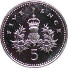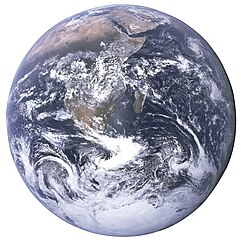Pi is an irational constant. For most uses 3.14 is precise enough.

Pi has been calculated to over 1 trillion digits but such calculations are entirely useless - 39 digits of pi would be enough to calculate the width of the universe to the precision of one atom. NASA use 15 digits.

## Polygon

A shape with straight sides.## Positive number

A number greater than zero.

All natural numbers are positive

## Power

eg 24 - 4 is the indice or power

## Precise

The number of decimal places that an answer can be quoted to.

## Precision

The number of decimal places that an answer can be quoted to.

## Prime

A number that has 2 factors - 1 and itself. 1 is not prime because it has only 1 factor. 0 is not prime either.

## Prism

A 3D shape with the same cross section all the way through it. If you could make it with a cookie cutter, it's a prism.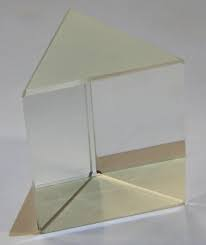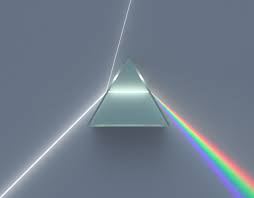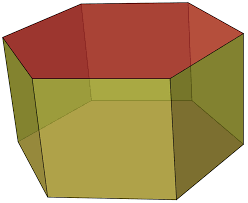## Probability

A measure of how likely an event is (eg rolling a 3 with dice)

## Product

The value you get when multiplying two values together. eg The product of 2 and 3 is 6 (=2x3)

## Pythagoras

A method for finding side lengths in a right angled triangle.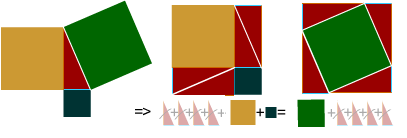The square on the hypotenuse is equal to the sum of the squares on the other two sides.

## Pythagorean triples

Pythagorean triples are sets of whole side lengths that define right angle triangles. These come up often and spotting one saves you having to calculate square roots eg:
3,4,5
5,12,13
8,15,17
7,24,25
20, 21, 29
12, 35, 37
9, 40, 41

They can be disguised by scaling. For example a 3,4,5 triangle may be scaled as 6,8,10, or 9,12,15, etc.

An equation where the highest indice is 2. All these equations are quadratic:
• y = x2 + 3x -5
• y = 4x2 + 3x -5
• y = x2 -5
• y = x2 + 3x

A polygon with 4 sides ('quad' is 4 - lateral is 'sides').## Quotient

A quotient is what is produced by dividing two numbers. This is often encountered as two numbers (ie a fraction or 12)

Less commonly a quotient is defined as the greatest whole number of times a divisor may be subtracted from a dividend—before making the remainder negative. For example, 7 ÷ 2 has a quotient of 3.

A shape is rotationally symmetrical if it still looks the same after turning it less than 1 complete rotation.

An equilateral triangle has 3 degrees of rotational symmetry, one for each place where the shape looks the same (including the full 360°).

Radians are a unit for measuring angles. Instead of dividing a circle into 360 equal parts (like degrees), radians divide a circle into 2 π (6.28) equal parts.

Why? Because 1 radian is the same distance as the radius of the circle

Most people prefer degrees but calculators often default to radians.The distance from the centre of a circle to its circumference.## Random sampling

A method of reducing a data set's size by choosing random data points. The assumption is that because the sample is chosen at random, that the random sample represents the whole data set.

There are lots of examples of this in opinion polls (where people say what they think) and in advertising (8 of 10 women agree their hair is silkier when they use ___). It would not be feasible to ask everyone.

## Range

The difference between the largest number and the smallest in a data set.

## Ratio

The factor by which one number varies with another.

## Rational

A decimal number that could be formed by dividing one whole number by another. You can often spot rational numbers spot because they either end (ie 0.125) or are recurring(eg 0.333333).

## Reciprocal

One divided by a number. The reciprocal of 2 is ½. The reciprocal of ½ is 2.

## Rectangle

A 4 sided shape where each angle is a right angle. A square is a special rectangle where all the sides are the same length.## Recurring

A decimal number which doesn't end but repeats after the decimal point (eg 1.3333... or 0.090909). It can be shown to be recurring by putting a dot over the recurring number, or all the recurring numbers. (eg. 1.3 or 0.09)

## Reflex angle

An angle greater than 180°.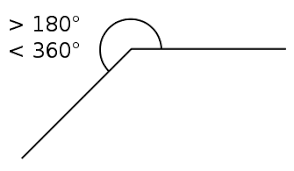## Regular

A polygon where all sides are equal and all angles are equal.## Relationship

The way one variable varies with another. For example y=3x+1 or y= 1x

## Remainder

The amount left over when a number can not be divided exactly.

eg 7 ÷ 4 = 1 remainder 3

## Rhombus

A quadrilateral with two lines of symetry, each from corner to corner.## Right angle

An angle of 90°## Right angled triangle

A triangle with a right angle.Right angled triangles are easy to work with because you can calculate side lengths with pythagoras and angles (and sides too) with simple trigonometry

## Rotation

To turn a shape a given angle and a given direction. To be precise, a centre of rotation has to be given.

## Rotational symmetry

A shape is rotationally symmetrical if it still looks the same after turning it less than 1 complete rotation.

An equilateral triangle has 3 degrees of rotational symmetry, one for each place where the shape looks the same (including the full 360°).

## Round number

A number that is exactly divisible by 10.

The more zeros a number has at the end, the rounder it is, eg:

• 872 is not round
• 870 is round
• 900 is rounder than 870
• 1000 is rounder than 900

## Rounding

To make a number easier to deal with but less precise by reducing the amount of significant figures. eg 3.14159 could be rounded as 3.142 or 3.14

## Running total

A total that is added to with each new number in the data set..

eg
 Dice throw Running total 2 2 3 5 4 9

## SI

The international system of units. It is wrtten SI rather than IS because it was named by the French: Système International.

## Scale factor

How many times greater or smaller the length and width or height) a scaled shape will be scaled by.

## Scalene

A Scalene triangle is one where no two sides are equal and no two angles are equal - although either one of these tests enough on its own because if the sides are all different lengths then no two angles can be the same. A right angled triangle can be a scalene but an iscosceles can't.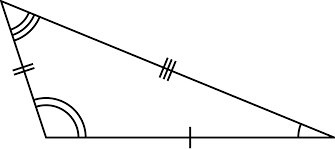## Scaling

To change the size of shape. To define it precisely, the Centre of enlargement needs to be deifined.

## Secant

A line passing through a circle but not through its centre.## Sector

A part of the area of a circle formed of two radii and part of the circumference between them.## Segment

An area between the circumference of a circle and a chord.## Septagon

A heptagon (also known as a septagon) is a 7 sided polygon (shape).Is a 50p coin a septagon (or heptagon)?## Sequence

A list of numbers which follow a pattern. eg
• 2,4,6,8...
• 50,45,40,35...
• 2,4,8,16,32....

## Significant figures

Each of the digits of a number that are used to express it to the required degree of accuracy, starting from the first non-zero digit.

## Simplify

To write an expression in its simplest (lowest) terms. eg:
• 24 = 12
• x2 + 3 + 3x2 + 2x - 7 -3x +2 = 4x2 - x - 2

## Solid

A closed 3D shape.

## Solve

Find a missing value. Frequently written as solve for the value to find. eg solve for x.

## Speed

How fast something is moving, most commonly in miles per hour (ie how many miles something would travel in 1 hour) in the UK.

If you can't remember the formula, think of the units. We say 30 miles per hour ie 30mileshour so speed is distance (miles)time (hours)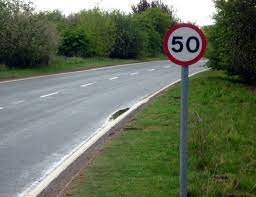## Square

A special rectangle where all the sides are the same length.## Square number

A number multiplied by itself. It's called square because if you draw what's happening, it's the area of a square with the given side length. Some square numbers include 1 (=1x1) 4 (=2x2), 9 (=3x3), 16(=4x4)

## Sum

The answer when adding values together. eg The sum of 3 and 2 is 5.

## Surface area

The total 'skin' area of a 3D shape.

## Symmetrical

A shape with 1 or more lines of symmetry.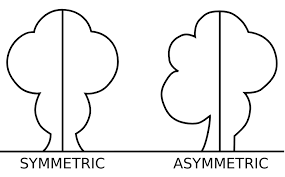## Symmetry

Where you can place a mirror on a shape and the reflected portion is the same as the half behind the mirror, that shape has symmetry. Where you have placed the mirror is a line of symmetry.## Tally

A way of recording a running total, by marking a number as groups of 5 (4 vertical lines and a horizontal one). It is useful for recording a running total.

IIII II (=7)

## Tangent

There are two uses of the word tangent in mathematics.
1. A straight line that touches a point on a curve wihtout passing through it. A tangent to a circle is, like the circumference it touches, perpendicular to the radius at that point.2. The function which maps a gradient on to an angle, shown here with the angle in radians.## Term

Part of an expression.

## The metric system

The international system of units. It is wrtten SI rather than IS because it was named by the French: Système International.

## Transformation

Reflections, rotations, translations and / or enlargements are all transformations.

## Translation

To slide a shape along the x or y axis.

## Trapezium

A quadrilateral where 2 sides are parallel to one another. The other 2 sides are not parallel to one another, or it would be a parallelogram.## Trapezoid

A quadrilateral where 2 sides are parallel to one another. The other 2 sides are not parallel to one another, or it would be a parallelogram.## Tree diagram

A list of the outcomes for a series of probable events. To work out the probability of 2 heads in 3 flips of a coin. you would start on the left wiht branches to heads and tails, and then each of those branches would branch again into heads and tails, and those branches would branch further.## Triangle

A 3 sided polygon.

## Triangular number

A series of numbers where the amount added increases by 1 with each number in the series.

## Trigonometry

A branch of maths concerned with angles and calculations involving angles.

## Unitary

1

Yes, it's that simple. Unitary, just means 1.

## Unitary fraction

A unitary fraction is a fraction that has a numerator of 1.

14 is a unitary fraction.

34 is a non-unitary fraction.

24 is also a non-unitary fraction, even though it can be simplified to a unitary fraction.

## Units

The scale of the quantity that the number expresses. For example, grams, metres, miles per hour, degrees, etc

## Upper quartile

The upper quartile is the highest 25% of data.

## Upper range

The largest value in a data set.

## Value

A numerical quantity.

## Variable

A letter in an expression. It may represent a fixed number (eg if 2x+1=5 can only be true is x =2), or a point anywhere along a line (eg y=3x+2)

## Vertex

A point where two straight lines meet.

## Vertical

An up-down orientation..## Volume

The amount of space an object takes up - like a 3D area.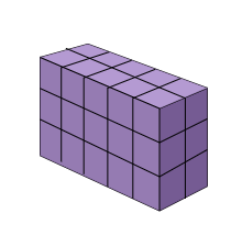## Week

Time period. There are 7 days in a week and roughly 52 weeks in a year.

## Wide

The side to side distance. eg a swimming pool may be 12m wide (or have a width of 12m).## Width

The side to side distance. eg a swimming pool may be 12m wide (or have a width of 12m).## X angles

Where two straight lines meet (forming an X), any pair of opposite angles will be equal.

eg A= B and C = D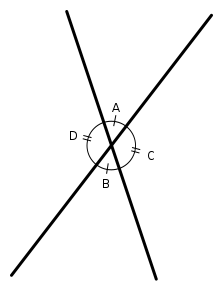## X-Axis

The horizontal axis. It is sometimes called y=0 because that is the formula of the line that lies along the x-axis.

## X-Intercept

The x-coordinate where a graph crosses the x-axis (eg y=0)

eg, the x intercept of the purple line is -1.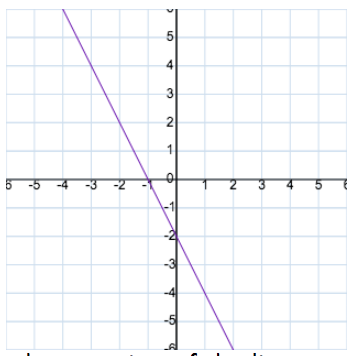## Y-Axis

The vertical axis. It is sometimes called x=0 because that is the formula of the line that lies along the y-axis.

## Y-Intercept

The y - coordinate of where a graph crosses the y-axis (eg x=0).

eg, the y intercept of the purple line is -2## Year

The amount of time it takes for the Earth to travel once around the sun. A year is 365 days (366 in a leap year), 12 months or roughly 52 weeks.

## Z-Axis

The third (normally depth) axis for a 3D space or graph.

## Z-angle

Where a line crosses two parallel lines, (forming a Z), the internal opposite angles will be equal.

In the diagram, where h and h| are parallel, α = β: